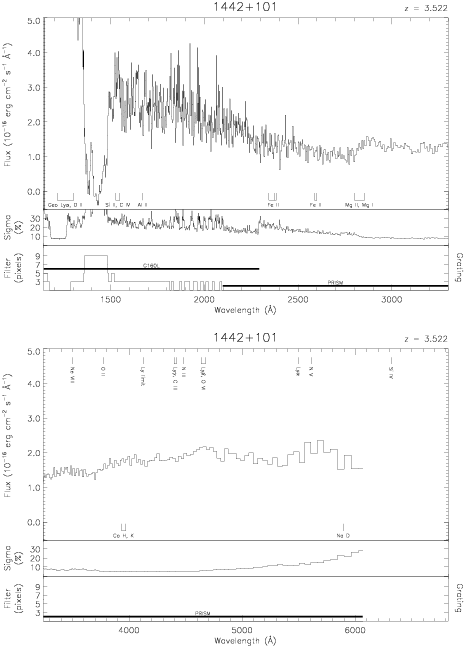Download dataset
TELESCOP= 'HST     '
INSTRUME= 'FOS     '
EQUINOX =               2000.0
DATE-OBS= '1991-06-06T07:43:31'
RA_PNT  =       221.3185579864
DEC_PNT =         9.9766306305
PA_PNT  =       307.7386000000
OBJECT  = '1442+101'
ROOTNM01= 'Y0LF0303T'
GRNDMD01= 'SPECTROSCOPY'
DETECT01= 'BLUE    '
APERID01= 'A-1     '
FGWAID01= 'L15     '
ROOTNM02= 'Y0LF0304T'
GRNDMD02= 'SPECTROSCOPY'
DETECT02= 'BLUE    '
APERID02= 'A-1     '
FGWAID02= 'L15     '
ROOTNM03= 'Y0LF0305T'
GRNDMD03= 'SPECTROSCOPY'
DETECT03= 'BLUE    '
APERID03= 'A-1     '
FGWAID03= 'L15     '
ROOTNM04= 'Y0LF0306T'
GRNDMD04= 'SPECTROSCOPY'
DETECT04= 'BLUE    '
APERID04= 'A-1     '
FGWAID04= 'PRI     '